Consider the following figure: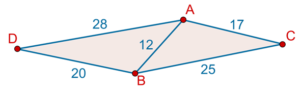How do we find the area of quadrilateral ACBD? Simple! We use Heron’s formula to calculate the areas of $$\Delta ABC$$ and $$\Delta ABC$$, and then add the two values to obtain the total area.

Area of $$\Delta ABC$$

\begin{align}&{s_{\Delta ABC}} = \frac{{12 + 25 + 17}}{2} = \frac{{54}}{2} = 27 & \,\,\,\\ &\Rightarrow {\rm{Are}}{{\rm{a}}_{\Delta ABC}} = \sqrt {27\left( {27 - 12} \right)\left( {27 - 25} \right)\\\left( {27 - 17} \right)} \\&\;\;\;\;\;\qquad\qquad\,\,\,= \sqrt {27 \times 15 \times 2 \times 10} \\ &\;\;\;\qquad\qquad\;\;\;\,= \sqrt {8100} \\ &\;\;\;\;\;\qquad\qquad\,\,\,= 90\;{\rm{sq}}{\rm{.}}\;{\rm{units}}\end{align}

Area of $$\Delta ABD$$

\begin{align}& {s_{\Delta ABD}} = \frac{{12 + 20 + 28}}{2} = \frac{{60}}{2} = 30 & \, & \,\,\\ &\Rightarrow {\rm{Are}}{{\rm{a}}_{\Delta ABD}} = \sqrt {30\left( {30 - 12} \right)\left({30 - 20} \right)\\\left( {30 - 28} \right)}\\ &\qquad\qquad\;\;\;\;\;\,\,\, = \sqrt {30 \times 18 \times 10 \times 2} \\ &\qquad\qquad\;\;\;\;\;\,\,\, = \sqrt {10800} \\ &\qquad\qquad\;\;\;\;\;\,\,\, = \sqrt {3600 \times 3} \\& \qquad\qquad\;\;\;\;\;\,\,\, = 60\sqrt 3 \;{\rm{sq}}{\rm{.}}\;{\rm{units}} \end{align}

Total Area

\begin{align}&{\rm{Are}}{{\rm{a}}_{ACBD}} = 90 + 60\sqrt 3 \\&\,\, \qquad \qquad = 30\left( {3 + 2\sqrt 3 } \right)\;{\rm{sq}}{\rm{.}}\;{\rm{units}}\end{align}

Note that if we were only given the lengths of the four sides, and were not given the length of the diagonal AB, we would have been unable to calculate the area. This is because if the length of AB is not fixed, there can be many (infinitely many) quadrilaterals with the same set of lengths for the four sides. Try to visualize this. However, once any diagonal length if fixed (along with the lengths of the sides), then the quadrilateral becomes unique, that is, there can be only one quadrilateral with given side lengths and a given diagonal length.

In principle, the area of any n-sided polygon can be calculated this way, if the lengths of the diagonals are known: just split the polygon into triangles, and use Heron’s formula to calculate the area of each triangle, and then add all the values. In the following figure, we have a 7-sided polygon, and the diagonal lengths are known (those shown in the figure). Thus, the area of each triangle can be calculated: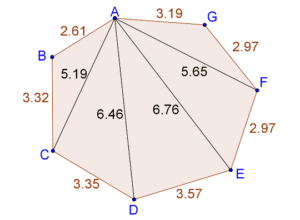As an exercise, use a calculator to approximate the area of this polygon using Heron’s formula.

Example 1: What is the area of the quadrilateral ABCD shown in the figure below?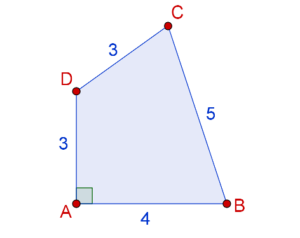Solution: Note that we have only been given the lengths of the four sides, but not the length of any diagonal. Fortunately, AB and AD are perpendicular, which means that the Pythagoras theorem can be used to calculate BD: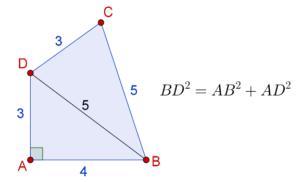Now, Heron’s formula can be applied on $$\Delta ABD$$ and $$\Delta BCD$$ separately.

Area of $$\Delta ABD$$\begin{align}&{s_{\Delta ABD}} = \frac{{4 + 5 + 3}}{2} = 6\\& \Rightarrow {A_{\Delta ABD}} = \sqrt {6 \times \left( {6 - 4} \right) \times \left( {6 - 5} \right) \times \left( {6 - 3} \right)} \\& \qquad \qquad \;\,\, = \sqrt {6 \times 2 \times 1 \times 3} \\& \qquad \qquad \;\; = 6\;{\rm{sq}}{\rm{.}}\;{\rm{units}}\\ &\qquad \qquad \,\,\,\,\,{\rm{or}}\\&\,\,\,\,\,{A_{\Delta ABD}} = \frac{1}{2} \times AB \times AD\\& \qquad \qquad = \frac{1}{2} \times 4 \times 3\\& \qquad \qquad = 6\;{\rm{sq}}{\rm{.}}\;{\rm{units}}\end{align}

Area of $$\Delta BCD$$

\begin{align}&{s_{\Delta BCD}} = \frac{{5 + 3 + 5}}{2} = \frac{{13}}{2}\\& \Rightarrow \qquad{A_{\Delta BCD}} = \sqrt {\frac{{13}}{2} \times \left( {\frac{{13}}{2} - 5} \right) \times \left( {\frac{{13}}{2} - 3} \right)\\ \times \left( {\frac{{13}}{2} - 5} \right)}\\& \qquad\qquad\qquad\,\,\, = \sqrt {\frac{{13}}{2} \times \frac{3}{2} \times \frac{7}{2} \times \frac{3}{2}}\\& \qquad\qquad\qquad\,\,\, = \frac{{3\sqrt {91} }}{4}\;{\rm{sq}}{\rm{.}}\;{\rm{units}}\\& \qquad\qquad\qquad\,\,\, \approx 7.15\;{\rm{sq}}{\rm{.}}\;{\rm{units}}\end{align}

Total Area

${A_{ABCD}} \approx 6 + 7.15 = 13.15\;{\rm{sq}}{\rm{.}}\;{\rm{units}}$

Example 2: ABCD is a trapezium with AB ∥ CD and the lengths of the sides as marked below: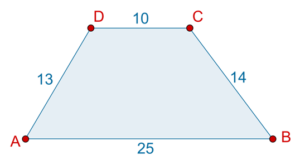What is the area of ABCD?

Solution: We have been given the lengths of the four sides, along with the additional fact that ABCD is a trapezium. This information must somehow be made use of. We will proceed as follows. Through C, draw CE ∥ AD as shown below: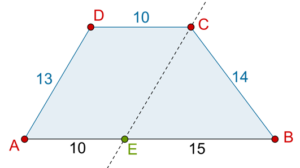Clearly, AECD is a parallelogram, because AE ∥ CD and AD ∥ CE. Thus, AE = 10, and so BE = 25 10 = 15. Also, CE = 13.

Now, let us use Heron’s formula to calculate the area of $$\Delta BEC$$.

\begin{align}& {s_{\Delta BEC}} = \frac{{15 + 13 + 14}}{2} = 21\\& \Rightarrow {A_{\Delta BEC}} =\;\;\; \sqrt { 21 \times \left( {21 - 15} \right) \times \left( {21 - 13} \right)\\ \times \left( {21 - 14} \right)} \\ &\qquad\qquad \,\,\, = \sqrt {21 \times 6 \times 8 \times 7} \\& \qquad\qquad \,\,\, = \sqrt {7056} \\& \qquad\qquad \;\; = 84\;{\rm{sq}}{\rm{.}}\;{\rm{units}} \end{align}

To calculate the area of parallelogram AECD, we need its height. Drop a perpendicular from C onto AB, as shown: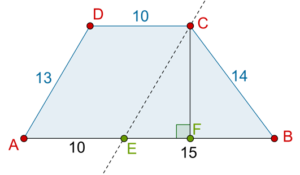CF, the distance between the parallels of the trapezium (and the parallelogram AECD also) can now be calculated as follows:

\begin{align}&{\rm{Area}}\left( {\Delta BEC} \right) = 84 = \frac{1}{2} \times BE \times CF\\ &\Rightarrow \qquad\qquad 84 = \frac{1}{2} \times 15 \times CF\\ &\Rightarrow \qquad\qquad CF = \frac{{2 \times 84}}{{15}} = 11.2\;{\rm{sq}}{\rm{.}}\;{\rm{units}}\end{align}

Thus, the area of the parallelogram AECD is:

$\begin{array}{l}{\rm{Area}}\left( {\Delta ECD} \right) = {\rm{Base}}\,\, \times {\rm{Height}}\\ \qquad\qquad \,\,\,\,\,\,\,\,\,\,\,\,\,\, = AE \times CF\\ \qquad\qquad \,\,\,\,\,\,\,\,\,\,\,\,\,\, = 10 \times 11.2\\ \qquad\qquad \,\,\,\,\,\,\,\,\,\,\,\,\,\, = 112\;{\rm{sq}}{\rm{.}}\;{\rm{units}}\end{array}$

Final, the (total) area of the trapezium ABCD is:

$\begin{array}{l} {\rm{Area}}\left( {\Delta BCD} \right) = 84 + 112\\ \,\,\,\,\,\,\,\,\,\,\,\,\,\,\qquad \qquad \,= 196\;{\rm{sq}}{\rm{.}}\;{\rm{units}} \end{array}$

grade 9 | Questions Set 2